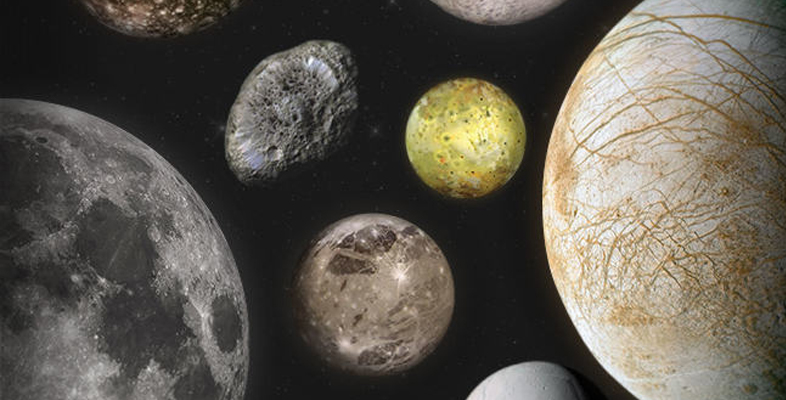Moons of our Solar System

Start this free course now. Just create an account and sign in. Enrol and complete the course for a free statement of participation or digital badge if available.

Free course

# 1.3 How small? How expensive?

Now you have a chance to weigh up some of the costs of sending objects into space. It’s a very basic non-scored activity. Don’t worry if you don’t know some of the answers. Take an educated guess.

## Activity 1 The costs of sending objects into space

Timing: Allow approximately 15 minutes.

What is the approximate cost of sending 1 kg into Earth orbit?

a.

$3000 b.$10 000

c.

$230 000 d.$600 000

b.

Well done.

How heavy was the Mars rover Curiosity?

a.

20 kg

b.

899 kg

c.

1000 kg

b.

Correct.

How much did the Curiosity rover cost to design, build and launch?

a.

$2 million b.$100 million

c.

$2.5 billion The correct answer is c. c. Correct. How much did the International Space Station cost? a.$10 billion

b.

$20 billion c.$150 billion

c.

Correct.

How much has the New Horizons mission to Pluto–Charon cost?

a.

$6 million b.$45 million

c.

$650 million d.$1 billion

c.

Correct.

MOONS_BOC_1